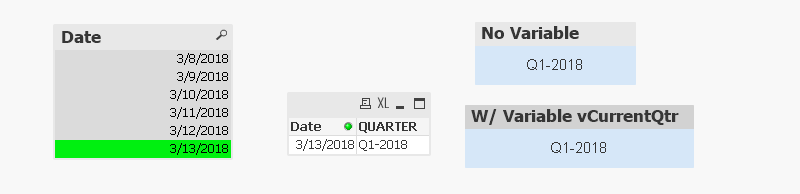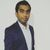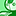# QlikView App Dev

Discussion Board for collaboration related to QlikView App Development.

Announcements
Customer & Partners, DEC. 9, 11 AM ET: Qlik Product & Strategy Roadmap Session: Data Analytics REGISTER NOW
cancel
Showing results for
Did you mean:Creator

## How to get the Current Quarter / Fiscal Period in Set Expression

Hi Folks,

I am new to Qlik and looking for ways to get the current quarter value in a set expression. I have following expression where I have hardcoded the values for the current quarter  (Q1-2018 below).

=Num(Round(Sum({<[Fiscal Period]={'Q1-2018'}>} DISTINCT  [Contractual TOV])/1000), '\$#,##0K')

how can I get rid of hardcoded value and make based on current date / system date? I need to get it in the same format as hardcoded here.

Can it be done thru a variable let's vCurrentQtr that is calculated out of some date function that can be reused in other places/expression?

Thanks,

Gaurav

1 Solution

Accepted SolutionsSpecialist III

Check this out.All the below calculations are based on current date.9 RepliesMaster

You mean to say..datewise sum?Creator
Author

I just want the CQ value Q1-2018 not to be a hardcoded one. It should come from some date functions... I don't know which function can help in it.Master

Depends on the fields in your model, do you have any quarter Field defined in model?

Can you share sample app?Creator
Author

No I just want it to based on current system date.Master

Try this

=Num(Round(Sum({<[Fiscal Period]={">=\$(=Num(QuarterStart(Max([Fiscal Period]))))<=\$(=Max([Fiscal Period]))"}>} DISTINCT  [Contractual TOV])/1000), '\$#,##0K')Specialist III

Check this out.All the below calculations are based on current date.Creator III

try with this,i hope it will help to you.

sum({<OrderDate = {">=\$(=Quarterstart(max(OrderDate)))<=\$(=max(OrderDate))"}>}Sales)MVP

May be this?

Num(Round(Sum({<[Fiscal Period]={'\$(=minstring({<[Fiscal year]={"\$(max([Fiscal year]))"}>} [Fiscal Period]))'}>} DISTINCT  [Contractual TOV])/1000), '\$#,##0K')

Before develop something, think If placed (The Right information | To the right people | At the Right time | In the Right place | With the Right context)Creator
Author

I think this is what I was looking for:

=Num(Round(Sum({<[Fiscal Period]={\$(=chr(39)& 'Q' & CEIL(MONTH(Today())/3) &'-'& YEAR(Today())&chr(39))}>} DISTINCT  [Contractual TOV])/1000), '\$#,##0K')

It fixes the issue and gives CQ based on system date.

Thanks,

GauravCommunity Browser